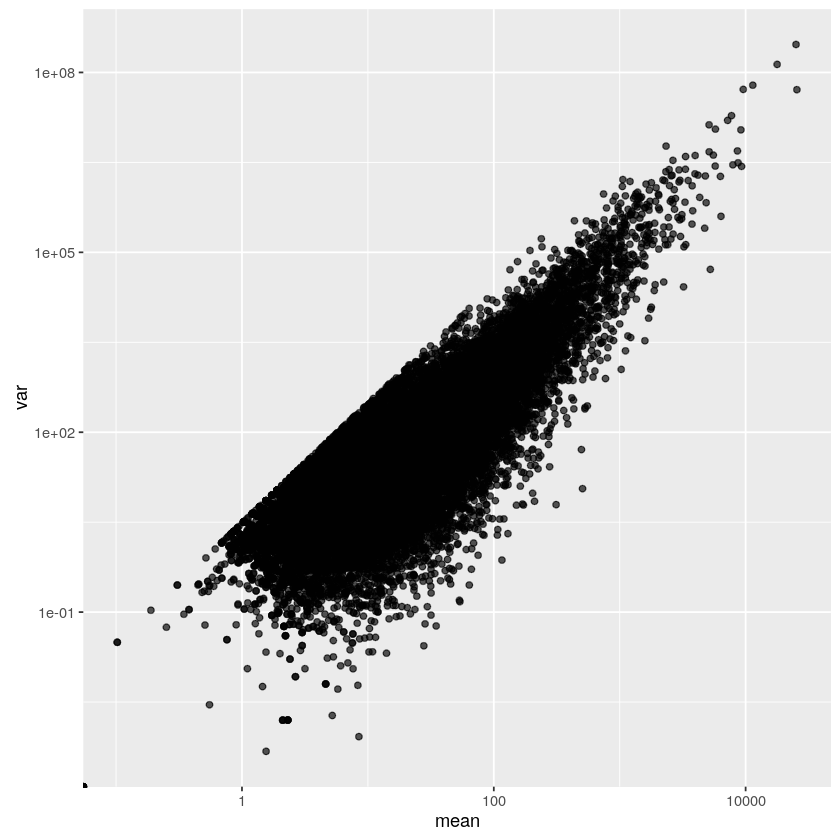# Mean-variance ceiling

Published on

Today I was playing with the count data from a small RNA-Seq experiment performed in Arabidopsis thaliana.

At some point, I decided to look at the mean-variance relationship for the fragment counts. As I said, the dataset is small; there are only 3 replicates per condition from which to estimate the variance. Moreover, each sample is from a different batch. I wasn’t expecting to see much.

But there was a pattern in the mean-variance plot that was impossible to miss.Mean-variance plot of counts per million, log-log scale

It is a nice straight line that many points lie on, but none dare to cross. A ceiling.

The ceiling looked mysterious at first, but then I found a simple explanation. The sample variance of $n$ numbers $a_1,\ldots,a_n$ can be written as

$\sigma^2=\frac{n}{n-1}\left(\frac1n\sum_{i=1}^n a_i^2-\mu^2\right),$

where $\mu$ is the sample mean. Thus,

$\frac{\sigma^2}{\mu^2}=\frac{\sum a_i^2}{(n-1)\mu^2}-\frac{n}{n-1}.$

For non-negative numbers, $n^2\mu^2=(\sum a_i)^2\geq \sum a_i^2$, and

$\frac{\sigma^2}{\mu^2}\leq\frac{n^2}{n-1}-\frac{n}{n-1}=n.$

This means that on a log-log plot, all points $(\mu,\sigma^2)$ lie on or below the line $y=2x+\log n$.

Moreover, the points that lie exactly on the line correspond to the samples where all $a_i$ but one are zero. In other words, those are gene-condition combinations where the gene’s transcripts were registered in a single replicate for that condition.